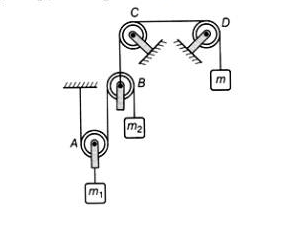For the situation shown in the figure, pulleys are light and smooth. Pulleys C and D are fixed, but A and B are movable. Find the value of m (in kg) if m is in equilibrium. (take, m1 = 2 kg and m2 =1 kg)

# For the situation shown in the figure, pulleys are light and smooth. Pulleys C and D are fixed, but A and B are movable. Find the value of m (in kg) if m is in equilibrium. (take, m1 = 2 kg and m2 =1 kg)Register to Get Free Mock Test and Study Material

+91

Verify OTP Code (required)

### Solution:

Let tension  of is T and tension of  ${m}_{1}$ is 2T

Let acceleration of is a and acceleration of  ${m}_{2}$ is 2a

Free body diagram (FBD) of m2

Free body diagram (FBD) of m1

From Eqs. (i)2 and (ii), we get

Free body diagram of mRegister to Get Free Mock Test and Study Material

+91

Verify OTP Code (required)